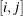### Initializing the Problem Data

The GA procedure offers great flexibility in how you initialize the problem data. Either you can read data from SAS data sets that are created from other SAS procedures and DATA steps, or you can initialize the data with programming statements.

In the PROC GA statement, you can specify up to five data sets to be read with the DATA= option, whereis a number from 1 to 5, that can be used to initialize parameters and data vectors applicable to the optimization problem. For example, weights and rewards for a knapsack problem could be stored in the variables `WEIGHT` and `REWARD` in a SAS data set. If you specify the data set with a DATA1= option, the arrays `WEIGHT` and `REWARD` are initialized at the start of the procedure and are available for computing the objective function and evaluating the constraints with program statements. You could store the number of items and weight limit constraint in another data set, as illustrated in the sample programming statements that follow:

```   data input1;
input weight reward;
datalines;
1    5
2    3
4    7
1    2
8    3
6    9
2    6
4    3
...
;
```
```   data input2;
input nitems limit;
datalines;
10  20
;

proc ga data1 = input1  /* creates arrays weight and reward */
data2 = input2; /* creates variables nitems and limit */

function objective( selected[*], reward[*], nitems);
array x /nosym;
call dynamic_array(x, nitems);
obj = 0;
do i=1 to nitems;
obj = obj + reward[x[i]];
end;
return(obj);
endsub;

[Other statements follow]
```

With these statements, the DATA1= option first establishes the arrays `weight` and `reward` from the data set `input1`, and the DATA2= option causes the variables `nitems` and `limit` to be created and initialized from the data set `input2`. The `reward` array and the `nitems` variable are then used in the objective function.

For convenience in initializing two-dimensional data such as matrices, the GA procedure provides you with the MATRIX= option, whereis a number from 1 to 5. A two-dimensional array is created within the GA procedure with the same name as the option, containing the numeric data in the specified data set. For example, a table of distances between cities for a traveling salesman problem could be stored as a SAS data set, and a MATRIX1= option specifying that data set would cause a two-dimensional array named `MATRIX1` to be created containing the data at the start of the GA procedure. This is illustrated in the following program:

```   data distance;
input d1-d10;
datalines;
0 5 3 1 2 ...
5 0 4 2 6 ...
3 4 0 1 3 ...
...
;
```
```   proc ga matrix1 = distance;
ncities = 10;
call SetEncoding('S10');
call SetObj('TSP','distances',matrix1);

[Other statements follow]
```

In this example, the data set `distance` is used to create a two-dimensional array `matrix1`, where `matrix1`is the distance from cityto city. The GA procedure provides a simple traveling salesman Problem (TSP) objective function, which is specified by the user with the SetObj call. The distances between locations are specified with the `distances` property of the TSP objective, which is set in the call to be `matrix1`. Note that when a MATRIX= option is used, the names of variables in the data set are not transferred to the GA procedure as they are with a DATA= option; only the numeric data are transferred.

You can also initialize problem data with programming statements. The programming statements in the GA procedure are executed before the optimization process begins. The variables created and initialized can be used and modified as the optimization progresses. The programming statement syntax is much like the SAS DATA step, with a few differences (see the section Syntax: GA Procedure). Special calls are described in the next sections that enable you to specify the objective function and genetic operators, and to monitor and control the optimization process. In the following program, a two-dimensional matrix is set up with programming statements to provide the distances for a 10-city symmetric traveling salesman problem, between locations specified in a SAS data set:

```   data positions;
input x y;
datalines;
100 230
50  20
150 100
...
;

proc ga data1 = positions;

call SetEncoding('S10');
ncities = 10;

array distance[10,10] /nosym;

do i = 1 to ncities;
do j = 1 to i;
distance[i,j] = sqrt((x[i]-x[j])**2 + (y[i] - y[j])**2);
distance[j,i] = distance[i,j];
end;
end;

call SetObj('TSP','distances', distance);
```

In this example, the DATA1= option creates arrays `x` and `y` containing the coordinates of the cities in an-grid, read in from the `positions` data set. An ARRAY programming statement creates a matrix of distances between cities, and the loops calculate Euclidean distances from the position data. The ARRAY statement is used to create internal data vectors and matrices. It is similar to the ARRAY statement used in the SAS DATA step, but the /NOSYM option is used in this example to set up the array without links to other variables. This option enables the array elements to be indexed more efficiently and the array to be passed efficiently to subroutines. You should use the /NOSYM option whenever you are creating an array that might be passed as an argument to a function or call routine.